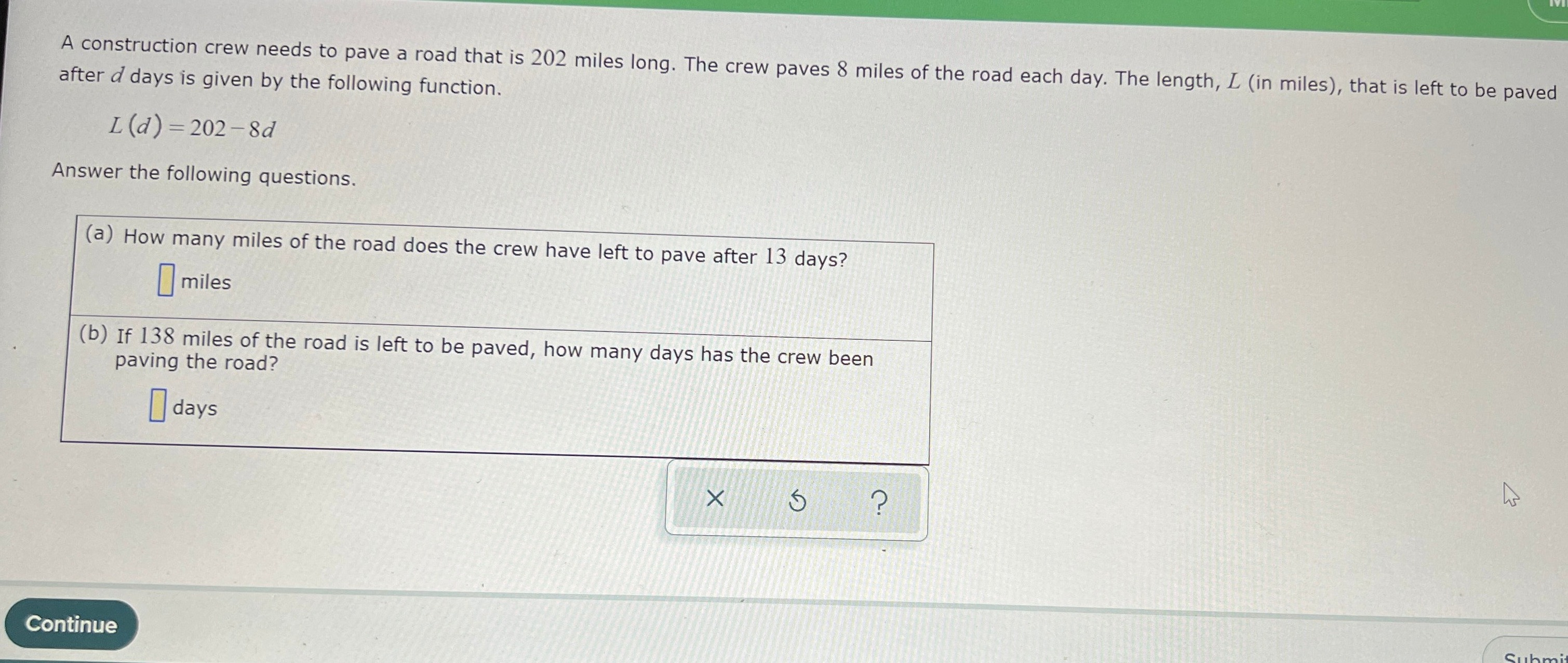### ¿Todavía tienes preguntas de matemáticas?

Pregunte a nuestros tutores expertos
Algebra
PreguntaA construction crew needs to pave a road that is $$202$$ miles long after $$d$$ days is given by the following function.

$$L ( d ) = 202 - 8 d$$

Answer the following questions. (a) How many miles of the road does the crew have left to (b) If $$138$$ miles of the road is left to be paved, how many d paving the road? Continue